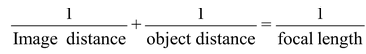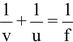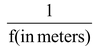# Mirror Formula

## MIRROR FORMULA

Let us first know about the terms used in the mirror formula of spherical mirrors.

• Object distance (u) : The distance of the object from the pole ‘P’ of the spherical mirror is called the object distance. It is denoted by the letter ‘u’
• Image Distance (v) : The distance of the image from the pole ‘P’ of the spherical mirror is called the image distance. It is denoted by the letter ‘v’.
• Focal length (f) : The distance of the principal focus (F) from the pole (P) of the spherical mirror is called the focal length. It is denoted by the letter ‘f ‘.

The relationship between the image distance (v), object distance (u) and focal length (f ) of a spherical mirror is known as the mirror formula. The Mirror formula can be written as :Symbolicallywhere the symbols have their usual meaning.

### POWER OF MIRROR:

A spherical mirror has infinite number of focus. Optical power of a mirror (in Dioptres)

= –